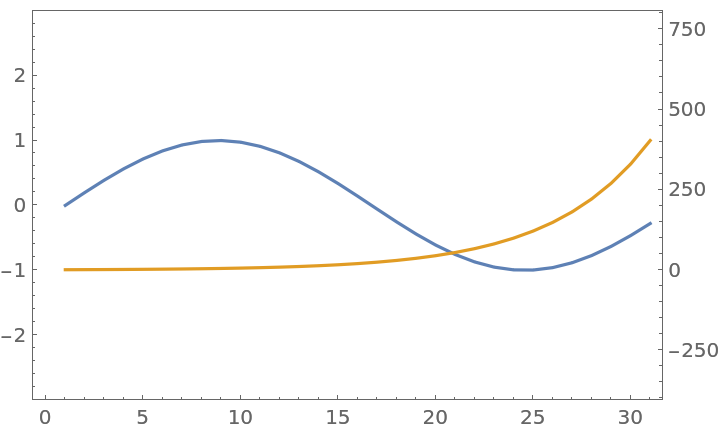#Function Repository Resource:

# MultipleAxesListPlot

A version of ListPlot that displays two lists of data with different y axes

Contributed by: Jon McLoone  |  Jon McLoone
 ResourceFunction["MultipleAxesListPlot"][{data1,data2}] generates a plot of the data with two y axes.

## Details and Options

In addition to all the options of ListPlot, the following options are supported:
 "SecondaryPlotRange" similar to PlotRange except applied only to the secondary y-axis "SecondaryAxisColor" choose the color of the secondary y-axis and ticks

## Examples

### Basic Examples (2)

Plot two datasets together but with different y-axes:

 In:=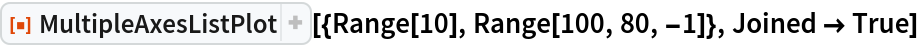Out=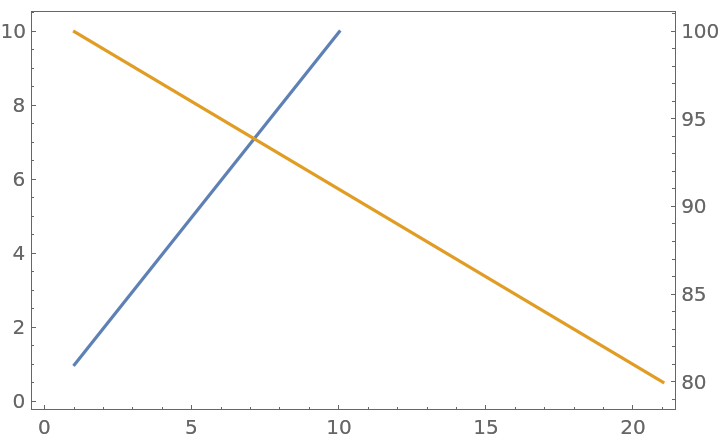Data can be a one-dimensional list or pairs of {x,y}-values:

 In:=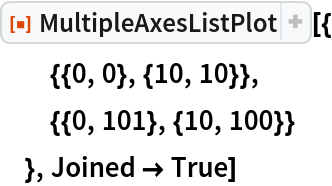Out=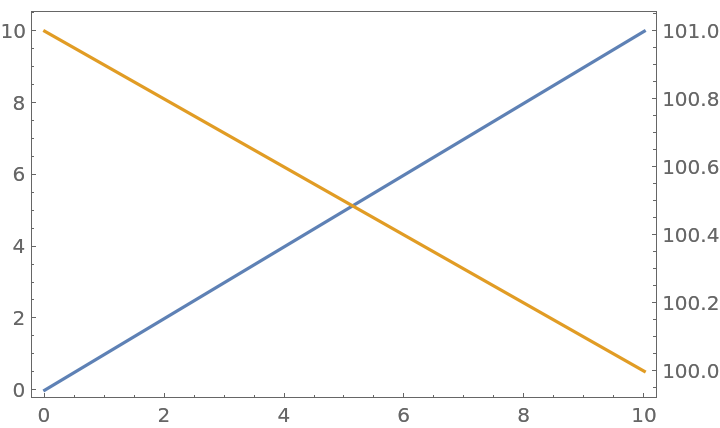### Options (3)

To make clearer which axis refers to which line, you can use "SecondaryAxesColor":

 In:=Out=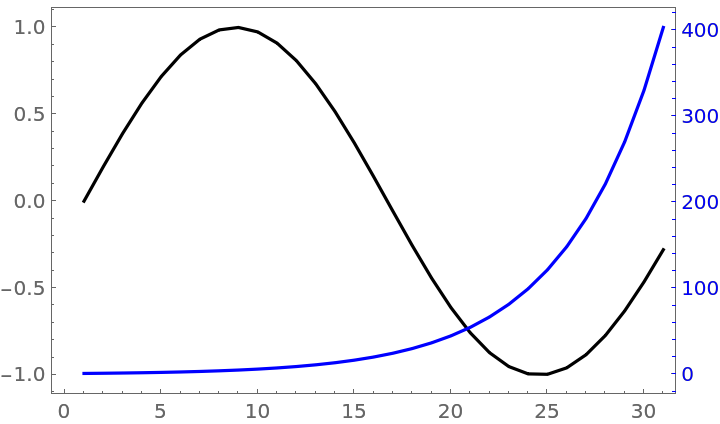You can control the plot ranges of the two lines using PlotRange and "SecondaryPlotRange":

 In:=Out=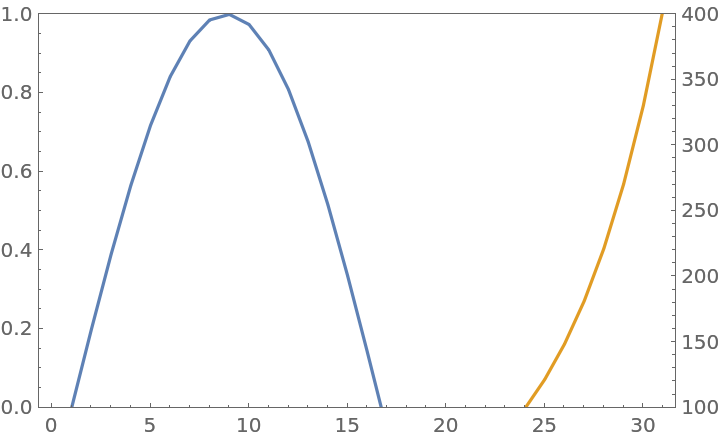PlotRangePadding applies to both datasets, but uses the coordinate system of the first dataset:

 In:=Out=